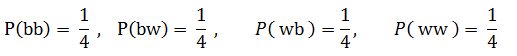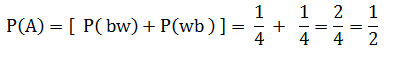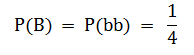# MA121 Introduction to Statistics coaching session 2.1.1 #9

9) Hi this is Asmita! I am a Student Success Coach and will be explaining some practice problems from the MA121 Introduction to Statistics course.Today we will review an assigned problem from Unit 2. In the “Homework Assessment” you were asked to solve this problem:

Assuming that each outcome is equally likely, find the probability of each event in Exercise 5.
We will use the concept of equally likely outcomes to find the probability of each outcomes.

Then we will approach this problem as follows:

1. Use the reference from exercise 5, and write down the given things.
2. Use the concept of probability of event to find the probability of event.
3. Find the required probability by plugging the individual probabilities.

By referring exercise 5, the sample space for the experiment of randomly drawing out, with replacement, two marbles in succession is S = {bb, bw, wb , ww }

where ‘b’ denotes black marble and ‘w’ denotes white marble.

“Equally likely outcomes” are the outcomes that have the same probability of occurring.
Sample space S has 4 outcomes. Since the whole sample space S is an event that is certain to occur, the sum of the probabilities of all the outcomes must be 1.
Since these four outcomes are equally likely, which must add up to 1, each outcome has probability 1/4. That is,a.

Step 1: Write the given things from exercise 5:
Event A: “At least one marble of each color is drawn”
Possible outcomes for event A = {bw, wb}

Step 2: Find the probability of event A:
To find the probability of event A, we find the sum of probability of individual outcomes in event A. Therefore,
P(A) = [ P( bw) + P(wb ) ]

Step 3: Plug the individual probabilitiesb.

Step 1: Write the given things from exercise 5:
Event B: “No white marble is drawn.”
Possible outcomes for event B = { bb }

Step 2: Find probability of event B:
To find probability of event B, we find the sum of probability of individual outcomes in event B. There is only one outcome, which is {bb}. Therefore,
P(B) = P(bb)

Step 3: Plug the individual probability:Conclusion:
a.The probability that at least one marble of each color is drawn is 1/2.
b.The probability that no white marble is drawn is 1/4.

In addition to this, I am also attaching the pdf document of the practice problem for your ready reference.
Question 9.pdf (304.6 KB)

Please let me know if you have any question on this problem, or on this topic generally. I will be here in the forum for the next hour.

I know this is an old post, are there still coaching sessions for MA121? Thanks